# mne.stats.spatio_temporal_cluster_test#

mne.stats.spatio_temporal_cluster_test(X, threshold=None, n_permutations=1024, tail=0, stat_fun=None, adjacency=None, n_jobs=None, seed=None, max_step=1, spatial_exclude=None, step_down_p=0, t_power=1, out_type='indices', check_disjoint=False, buffer_size=1000, verbose=None)[source]#

Non-parametric cluster-level test for spatio-temporal data.

This function provides a convenient wrapper for `mne.stats.permutation_cluster_test()`, for use with data organized in the form (observations × time × space), (observations × time × space), or optionally (observations × time × frequencies × space). For more information, see .

Parameters:
X`list` of `array`, shape (n_observations, p[, q], n_vertices)

The data to be clustered. Each array in `X` should contain the observations for one group. The first dimension of each array is the number of observations from that group (and may vary between groups). The second, and optionally third, dimensions correspond to the time or time-frequency data. And, the last dimension should be spatial. All dimensions except the first should match across all groups.

threshold

The so-called “cluster forming threshold” in the form of a test statistic (note: this is not an alpha level / “p-value”). If numeric, vertices with data values more extreme than `threshold` will be used to form clusters. If `None`, an F-threshold will be chosen automatically that corresponds to a p-value of 0.05 for the given number of observations (only valid when using an F-statistic). If `threshold` is a `dict` (with keys `'start'` and `'step'`) then threshold-free cluster enhancement (TFCE) will be used (see the TFCE example and ). See Notes for an example on how to compute a threshold based on a particular p-value for one-tailed or two-tailed tests.

n_permutations`int`

The number of permutations to compute.

tail`int`

If tail is 1, the statistic is thresholded above threshold. If tail is -1, the statistic is thresholded below threshold. If tail is 0, the statistic is thresholded on both sides of the distribution.

stat_fun

Function called to calculate the test statistic. Must accept 1D-array as input and return a 1D array. If `None` (the default), uses `mne.stats.f_oneway`.

Defines adjacency between locations in the data, where “locations” can be spatial vertices, frequency bins, time points, etc. For spatial vertices, see: `mne.channels.find_ch_adjacency()`. If `False`, assumes no adjacency (each location is treated as independent and unconnected). If `None`, a regular lattice adjacency is assumed, connecting each spatial location to its neighbor(s) along the last dimension of each group `X[k]`. If `adjacency` is a matrix, it is assumed to be symmetric (only the upper triangular half is used) and must be square with dimension equal to `X[k].shape[-1]` (n_vertices) or `X[k].shape[-1] * X[k].shape[-2]` (n_times * n_vertices) or (optionally) `X[k].shape[-1] * X[k].shape[-2] * X[k].shape[-3]` (n_times * n_freqs * n_vertices). If spatial adjacency is uniform in time, it is recommended to use a square matrix with dimension `X[k].shape[-1]` (n_vertices) to save memory and computation, and to use `max_step` to define the extent of temporal adjacency to consider when clustering.

n_jobs

The number of jobs to run in parallel. If `-1`, it is set to the number of CPU cores. Requires the `joblib` package. `None` (default) is a marker for ‘unset’ that will be interpreted as `n_jobs=1` (sequential execution) unless the call is performed under a `joblib.parallel_backend()` context manager that sets another value for `n_jobs`.

seed`None` | `int` | instance of `RandomState`

A seed for the NumPy random number generator (RNG). If `None` (default), the seed will be obtained from the operating system (see `RandomState` for details), meaning it will most likely produce different output every time this function or method is run. To achieve reproducible results, pass a value here to explicitly initialize the RNG with a defined state.

max_step`int`

Maximum distance between samples along the second axis of `X` to be considered adjacent (typically the second axis is the “time” dimension). Only used when `adjacency` has shape (n_vertices, n_vertices), that is, when adjacency is only specified for sensors (e.g., via `mne.channels.find_ch_adjacency()`), and not via sensors and further dimensions such as time points (e.g., via an additional call of `mne.stats.combine_adjacency()`).

spatial_exclude

List of spatial indices to exclude from clustering.

step_down_p`float`

To perform a step-down-in-jumps test, pass a p-value for clusters to exclude from each successive iteration. Default is zero, perform no step-down test (since no clusters will be smaller than this value). Setting this to a reasonable value, e.g. 0.05, can increase sensitivity but costs computation time.

t_power`float`

Power to raise the statistical values (usually F-values) by before summing (sign will be retained). Note that `t_power=0` will give a count of locations in each cluster, `t_power=1` will weight each location by its statistical score.

Output format of clusters within a list. If `'mask'`, returns a list of boolean arrays, each with the same shape as the input data (or slices if the shape is 1D and adjacency is None), with `True` values indicating locations that are part of a cluster. If `'indices'`, returns a list of tuple of ndarray, where each ndarray contains the indices of locations that together form the given cluster along the given dimension. Note that for large datasets, `'indices'` may use far less memory than `'mask'`. Default is `'indices'`.

check_disjoint`bool`

Whether to check if the connectivity matrix can be separated into disjoint sets before clustering. This may lead to faster clustering, especially if the second dimension of `X` (usually the “time” dimension) is large.

buffer_size

Block size to use when computing test statistics. This can significantly reduce memory usage when `n_jobs > 1` and memory sharing between processes is enabled (see `mne.set_cache_dir()`), because `X` will be shared between processes and each process only needs to allocate space for a small block of locations at a time.

verbose

Control verbosity of the logging output. If `None`, use the default verbosity level. See the logging documentation and `mne.verbose()` for details. Should only be passed as a keyword argument.

Returns:
F_obs`array`, shape (p[, q], n_vertices)

Statistic (F by default) observed for all variables.

clusters`list`

List type defined by out_type above.

cluster_pv: `array`

P-value for each cluster.

H0`array`, shape (n_permutations,)

Max cluster level stats observed under permutation.

Notes

For computing a `threshold` based on a p-value, use the conversion from `scipy.stats.rv_continuous.ppf()`:

```pval = 0.001  # arbitrary
dfn = n_conditions - 1  # degrees of freedom numerator
dfd = n_observations - n_conditions  # degrees of freedom denominator
thresh = scipy.stats.f.ppf(1 - pval, dfn=dfn, dfd=dfd)  # F distribution
```

References

## Examples using `mne.stats.spatio_temporal_cluster_test`#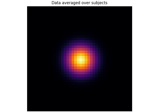Statistical inference

Statistical inference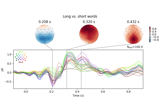Visualising statistical significance thresholds on EEG data

Visualising statistical significance thresholds on EEG data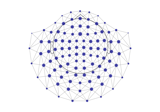Spatiotemporal permutation F-test on full sensor data

Spatiotemporal permutation F-test on full sensor data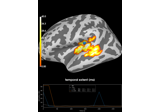2 samples permutation test on source data with spatio-temporal clustering

2 samples permutation test on source data with spatio-temporal clustering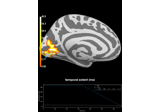Repeated measures ANOVA on source data with spatio-temporal clustering

Repeated measures ANOVA on source data with spatio-temporal clustering